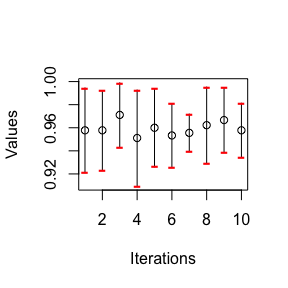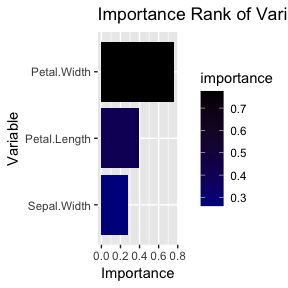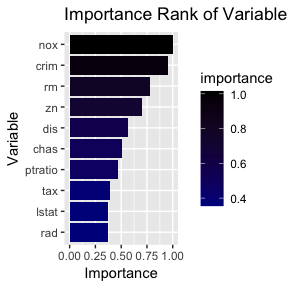# Introduction

Feature selection can be loosely defined as finding an optimal subset of available features in a dataset that are asssociated with the response variable. There are three broad categories of featue selection methods: filter methods, wrapper methods, and embedded methods. In the vignette, we introduce the Simultaneous Perturbation Stochastic Approximation for Feature Selection and Ranking (SPSA-FSR) Algorithm as one of the wrapper methods and how to use the spFSR package which implements this algorithm. The spFSR package is built upon the works by Aksakalli and Malekipirbazari (2016) <arXiv:1508.07630> and Yenice et al. (2018) <arXiv:1804.05589>.

As the spFSR package depends upon the mlr package, we shall follow the mlr workflow to define a learner and a task. The spFSR package supports classification and regression problems. We show how to perform feature selection with the spFSR package with two applications - one classification problem and one regression problem. The spFSR package does not support unsupervised learning (such as clustering), cost-sensitive classification, and survival analysis.

# SPSA-FSR Algorithm

Let X be an $$n \times p$$ data matrix of $$p$$ features and $$n$$ observations whereas $$Y$$ denotes the $$n \times 1$$ response vector consitute as the dataset. Let $$X:= \{ X_1, X_2, ....X_p \}$$ denote the feature set where $$X_j$$ represents the $$j^{th}$$ feature in. For a nonempty subset $$X' \subset X$$, we define $$\mathcal{L}_{C}(X', Y)$$ as the true value of performance criterion of a wrapper classifier (the model) $$C$$ on the dataset. As $$\mathcal{L}_{C}$$ is not known, we train the classifier $$C$$ and compute the error rate, which is denoted by $$y_C(X', Y)$$. Therefore, $$y_C = \mathcal{L}_C + \varepsilon$$. The wrapper feature selection problem is defined as determining the non-empty feature set $$X^{*}$$:

$X^{*} := \arg \min_{X' \subset X}y_C(X', Y)$

It is a stochastic optimisation problem because the functional form of $$Y$$ is unknown and can be estmated using stochastic optimisation algorithms, including the Simultaneous Perturbation Stochastic Approximation (SPSA). Introduced by Spall (1992), the SPSA is a pseudo-gradient descent stochastic optimisation algorithm. It starts with a random solution (vector) and moves toward the optimal solution in successive iterations in which the current solution is perturbed simultaneously by random offsets generated from a specified probability distribution. The SPSA-FSR algorithm is therefore an application of the SPSA for feature selection.

In the context of SPSA, given $$w \in D \subset \mathbb{R}^{p}$$, the loss function is given as:

$\mathcal{L}(w): D \mapsto \mathbb{R}$

where its functional form is unknown but one can observe noisy measurement:

$y(w) := \mathcal{L}(w) + \varepsilon(w)$

where $$\varepsilon$$ is the noise. Let $$g(w)$$ denote the gradient of $$\mathcal{L}$$:

$g(w): = \nabla \mathcal{L} = \frac{\partial \mathcal{L} }{\partial w}$

The SPSA-FSR algorithm uses a binary version of the SPSA where $$w \in \mathbb{Z}^{p}$$. Hence, the loss function becomes $$\mathcal{L}: \{0,1\}^{p} \mapsto \mathbb{R}$$. The SPSA-FSR algorithm starts with an initial solution $$\hat{w}_0$$ and iterates following the recursion below in search for a local minima $$w^{*}$$:

$\hat{w}_{k+1} := \hat{w}_{k} - a_k \hat{g}(\hat{w}_{k})$

where:

• $$a_{k}$$ is an iteration gain sequence; $$a_{k} \geq 0$$;
• $$\hat{g}(\hat{w}_{k})$$ is the approximate gradient at $$\hat{w}_{k}$$.

Let $$\Delta_k \in \mathbb{R}^p$$ be a simultaneous perturbation vector at iteration $$k$$. Spall (1992) suggests to imposes certain regularity conditions on $$\Delta_k$$:

• The components of $$\Delta_{k}$$ must be mutually independent;
• Each component of $$\Delta_{k}$$ must be generated from a symmetric zero mean probability distribution;
• The distribution must have a finite inverse;
• In addition, $$\{ \Delta_k\}_{k=1}$$ must be a mutually independent sequence which is independent of $$\hat{w}_0, \hat{w}_1,...\hat{w}_k$$.

The finite inverse requirement basically precludes $$\Delta_k$$ from uniform or normal distributions. A good candidate is a symmetric zero mean Bernoulli distribution, say $$\pm 1$$ with 0.5 probability. The SPSA-FSR algorithm “perturbs” the current iterate $$\hat{w}_k$$ by an amount of $$c_k \Delta_k$$ in each direction of $$\hat{w}_k + c_k \Delta_k$$ and $$\hat{w}_k - c_k \Delta_k$$ respectively. Hence, the simultaneous perturbations around $$\hat{w}_{k}$$ are defined as:

$\hat{w}^{\pm}_k := \hat{w}_{k} \pm c_k \Delta_k$

where $$c_k$$ is a nonnegative gradient gain sequence. The noisy measurements of $$\hat{w}^{\pm}_k$$ at iteration $$k$$ become:

$y^{+}_k:=\mathcal{L}(\hat{w}_k + c_k \Delta_k) + \varepsilon_{k}^{+} \\ y^{-}_k:=\mathcal{L}(\hat{w}_k - c_k \Delta_k) + \varepsilon_{k}^{-}$

where $$\mathbb{E}( \varepsilon_{k}^{+} - \varepsilon_{k}^{-}|\hat{w}_0, \hat{w}_1,...\hat{w}_k, \Delta_k) = 0 \forall k$$. At each iteration, $$\hat{w}_k^{\pm}$$ are bounded and rounded before $$y_k^{\pm}$$ are evaluated. Therefore, $$\hat{g}_k$$ is computed as:

$\hat{g}_k(\hat{w}_k):=\bigg[ \frac{y^{+}_k-y^{-}_k}{w^{+}_{k1}-w^{-}_{k1}},...,\frac{y^{+}_k-y^{-}_k}{w^{+}_{kp}-w^{-}_{kp}} \bigg]^{T} = \bigg[ \frac{y^{+}_k-y^{-}_k}{2c_k \Delta_{k1}},...,\frac{y^{+}_k-y^{-}_k}{2c_k \Delta_{kp}} \bigg]^{T} = \frac{y^{+}_k-y^{-}_k}{2c_k}[\Delta_{k1}^{-1},...,\Delta_{kp}^{-1}]^{T}$

For convergence, Spall (1992) proposes the iteration and gradient gain sequences to be:

• $$a_k := \frac{a}{(A+k)^{\alpha}}$$
• $$c_k := \frac{c}{\gamma^{k}}$$

$$A$$, $$a$$, $$\alpha$$, $$c$$ and $$\gamma$$ are pre-defined; these parameters must be fine-tuned properly. In the SPSA-FSR algorithm, we set $$\gamma = 1$$ so that $$c_k$$ is a constant. The detail of fine-tuning values are found in Aksakalli and Malekipirbazari (2016). Yenice et al. (2018) propose a nonmonotone Barzilai-Borwein method (Barzilai and Borwein 1988) to smooth the gain via

$\hat{b}_k = \frac{\sum_{n=k-t}^k{\hat{a}_{n}^{'}}}{t+1}$

The role of $$\hat{b}_k$$ is to eliminate the irrational fluctuations in the gains and ensure the stability of the SPSA-FSR algorithm. It averages the gains at the current and last two iterations, i.e. $$t=2$$. Gain smoothing results in a decrease in convergence time. Due to its stochastic nature and noisy measurements, the gradients $$\hat{g}(\hat{w})$$ can be approximately wrongly and hence distort the convergence direction in SPSA-FRS algorithm. To mitigate such side effect, the current and the previous $$m$$ gradients are averaged as a gradient estimate at the current iteration:

$\hat{g_k}(\hat{w_k}) = \frac{\sum_{n=k-m}^k{\hat{g_{n}}(\hat{w_{k}})}}{m+1}$

The SPSA-FSR algorithm does not have automatic stopping rules. So, we can specify a maximum number of iterations as a stopping criterion. The SPSA-FSR algorithm is summarised as:

• Inputs: $$\hat{w}_0$$, $$\{a_k\}$$, $$c$$, and maximum number of iterations $$M$$.
• Initialise $$k=0$$
• While $$(k < M)$$, do:
1. Generate $$\Delta_{k, j} \sim \text{Bernoulli}(-1, +1)$$ with $$\mathbb{P}(\Delta_{k, j}=1) = \mathbb{P}(\Delta_{k, j}=-1) = 0.5$$ for $$j=1,..p$$
2. Compute $$\hat{w}^{\pm}_k:=\hat{w}_{k} \pm c \Delta_k$$
3. Bound and then round:
• $$\hat{w}^{\pm}_k \mapsto B(\hat{w}^{\pm}_k)$$ where $$B( \bullet)$$ is the component-wise $$[0,1]$$ operator.
• $$B(\hat{w}^{\pm}_k) \mapsto R(\hat{w}^{\pm}_k)$$ where $$R( \bullet)$$ is the component-wise rounding operator.
1. Evaluate $$y^{\pm}_k:=\mathcal{L}(\hat{w}_k + c_k \Delta_k) \pm \varepsilon_{k}^{+}$$
2. Compute the gradient estimate:

$\hat{g}_k(\hat{w}_k):=\bigg( \frac{y^{+}_k-y^{-}_k}{2c}\bigg)[\Delta_{k1}^{-1},...,\Delta_{kp}^{-1}]^{T}$

1. Update $$\hat{w}^{\pm}_k := \hat{w}_{k} \pm c_k \Delta_k$$
• Round $$\hat{w}_M$$ if necessary.
• Output: Estimate of solution vector $$\hat{w}^{*}:=\hat{w}_M$$.

# Installation

The spFSR package can be installed from CRAN as follow:

install.packages("spFSR")

If it is installed manually from an archive (tar.gz), then the following dependency and imported packages must be installed first:

if(!require("mlr") ){ install.packages("mlr") }                 # mlr (>= 2.11)
if(!require("parallelMap") ){ install.packages("parallelMap") } # parallelMap (>= 1.3)
if(!require("parallel") ){ install.packages("parallel")}        # parallel (>= 3.4.2)
if(!require("tictoc") ){ install.packages("tictoc") }           # tictoc (>= 1.0)
if(!require("ggplot2") ){ install.packages("ggplot2") }         # tictoc (>= 1.0)
if(!require('spFSR') ){
install.packages('spFSR_1.0.0.tar.gz', repos = NULL)
}

The mlr depends on other packages. Although only some are utilised in the spFSR, it is highly recommended to install the suggested packages:

ada, adabag, bartMachine, batchtools, brnn, bst, C50, care, caret (>= 6.0-57), class, clue, cluster, clusterSim (>= 0.44-5), clValid, cmaes, CoxBoost, crs, Cubist, deepnet, DiceKriging, DiceOptim, DiscriMiner, e1071, earth, elasticnet, elmNN, emoa, evtree, extraTrees, flare, fields, FNN, fpc, frbs, FSelector, gbm, GenSA, ggvis, glmnet, h2o (>= 3.6.0.8), GPfit, Hmisc, ipred, irace (>= 2.0), kernlab, kknn, klaR, knitr, kohonen, laGP, LiblineaR, lqa, MASS, mboost, mco, mda, mlbench, mldr, mlrMBO, modeltools, mRMRe, nnet, nodeHarvest (>= 0.7-3), neuralnet, numDeriv, pamr, party, penalized (>= 0.9-47), pls, PMCMR (>= 4.1), pROC (>= 1.8), randomForest, randomForestSRC (>= 2.2.0), ranger (>= 0.6.0), RCurl, Rfast, rFerns, rjson, rknn, rmarkdown, robustbase, ROCR, rotationForest, rpart, RRF, rrlda, rsm, RSNNS, RWeka, sda, shiny, smoof, sparsediscrim, sparseLDA, stepPlr, SwarmSVM, svglite, testthat, tgp, TH.data, xgboost (>= 0.6-2), XML

To see why, say we would like to apply k-nearest neighbour (knn) on a classification problem. In the mlr, this can be done by defining a learner as mlr::makeLearner("classif.knn", k = 5) in R. Note that "classif.knn" is called from the class package via mlr. So it the class package has not been installed, this learner cannot be defined. To get the full list of learners from the mlr package, see listLearners() for more details.

# Applications

## Classification Problem

### Dataset

Using the classical iris data, the goal is to choose 3 of 4 features (Sepal.Length, Sepal.Width, Petal.Length, and Petal.Width) that give the highest mean accuracy rate in predicting Species.

data(iris)
head(iris)
#>   Sepal.Length Sepal.Width Petal.Length Petal.Width Species
#> 1          5.1         3.5          1.4         0.2  setosa
#> 2          4.9         3.0          1.4         0.2  setosa
#> 3          4.7         3.2          1.3         0.2  setosa
#> 4          4.6         3.1          1.5         0.2  setosa
#> 5          5.0         3.6          1.4         0.2  setosa
#> 6          5.4         3.9          1.7         0.4  setosa

### Define Task and Wrapper

After loading the mlr package, we create a wrapper which is a knn learner with $$k=5$$. Then, we make a classification task by specifying Species as the response or target variable we would like to predict. Lastly, we specify acc (accuracy) to evaluate the wrapper’s performance.

library(mlr)
#> Loading required package: ParamHelpers
#> Warning: replacing previous import 'BBmisc::isFALSE' by
#> 'backports::isFALSE' when loading 'mlr'
knnWrapper    <- makeLearner("classif.knn", k = 5)
classifTask   <- makeClassifTask(data = iris, target = "Species")
perf.measure  <- acc

### Select features using spFeatureSelection

The spFeatureSelection function requires four main arguments:

• task: a task object created using mlr package. In this example, task = classifTask
• wrapper: a Learner object created using mlr package. In this example, it is wrapper = knnWrapper
• measure: a performance measure supported by task; here, measure = perf.measure
• num.features.selected: number of features to be selected. In this example, we aim to choose three features (num.features.selected = 3).

In addition, due to the stochastic nature of the SPSA-FSR algorithm, we recommend user to run it multiple iterations by specifying iters.max in spFeatureSelection. The default value of iters.max is 25. For illustration, we shall run up to 10 iterations by specifying iters.max = 10. To speed up, user can specify how many processor cores to run the algorithm. The default value is 2 or the minimum core available on the computer. In this example, we apply a single core (num.cores = 1).

library(spFSR)
#> Loading required package: parallelMap
#> Loading required package: parallel
#> Loading required package: tictoc
set.seed(123)
spsaMod <- spFeatureSelection(
task = classifTask,
wrapper = knnWrapper,
measure = perf.measure ,
num.features.selected = 3,
iters.max = 10,
num.cores = 1)
#> SPSA-FSR begins:
#> Wrapper = knn
#> Measure = acc
#> Number of selected features = 3
#>
#> iter  value   st.dev  num.ft  best.value
#> 1     0.95778 0.03666 3       0.95778 *
#> 2     0.95778 0.03443 3       0.95778
#> 3     0.97111 0.02779 3       0.97111 *
#> 4     0.95111 0.04153 3       0.97111
#> 5     0.96    0.03381 3       0.97111
#> 6     0.95333 0.0276  3       0.97111
#> 7     0.95556 0.01627 3       0.97111
#> 8     0.96222 0.03301 3       0.97111
#> 9     0.96667 0.02817 3       0.97111
#> 10    0.95778 0.02346 3       0.97111
#>
#> Best iteration = 3
#> Number of selected features = 3
#> Best measure value = 0.97111
#> Std. dev. of best measure = 0.02779
#> Run time = 0.1 minutes.

The output above shows that the result produced by spFeatureSelection. At each iteration (iter), it shows mean accuracy rate (value) and its standard deviation (st.dev) on three features (num.fit = 3). The best.value represents the best mean accuracy rate produced by spFeatureSelection. At the first iteration (iter = 1), the best mean accuracy rate is 0.96 and it is denoted by ’*’. At the second iteration, the mean accuracy rate is lower and hence the accuracy rate from the first iteration remains as the best value. At the third iteration, the accuracy rate improves to 0.97111 which is higher the previous best value. The accuracy rate of the third iteration therefore becomes the best value.

### Generic methods

The spFSR package supports three S3 generic methods: print, summary, and plot. The usages of print and summary are quite straighforward. The summary return the following information:

summary(spsaMod)
#> $target #>  "Species" #> #>$importance
#>       features importance
#> 1  Petal.Width    0.76373
#> 2 Petal.Length    0.39640
#> 3  Sepal.Width    0.27386
#>
#> $nfeatures #>  3 #> #>$niters
#>  10
#>
#> $name #>  "k-Nearest Neighbor" #> #>$best.iter
#>  3
#>
#> $best.value #>  0.97111 #> #>$best.std
#>  0.02779
#>
#> attr(,"class")
#>  "summary.spFSR"

We can produce a scatterplot of mean accuracy rate at each iteration by calling the plot function on spsaMod. We can also add an error bar of $$\pm 1$$ standard deviation around the mean accuracy rate at each iteration by specifying errorBar = TRUE. Other graphical parameters such as pch, type, ylab, and col are allowed.

plot(spsaMod, errorBar = TRUE)### Other functions

The spFSR package has:

• getImportance which returns the importance ranks of best performing features as a data.frame object
• plotImportance which plots the importance ranks of best performing features
• getBestModel which returns the trained or wrapped model based on the set of best performing features.
getImportance(spsaMod)
#>       features importance
#> 1  Petal.Width    0.76373
#> 2 Petal.Length    0.39640
#> 3  Sepal.Width    0.27386
plotImportance(spsaMod)The vertical bar chart generated by plotImportance shows that Petal.Width is the most important feature. We can obtain the best performing model by calling getBestModel.

bestMod <- getBestModel(spsaMod)

bestMod is an object of WrapperModel class from the mlr package.

class(bestMod)
#>  "WrappedModel"

It inherits all methods of this class including predict. The predict function can be used to predict out-of-sample data using setting new.data to a test data. It can be also used to return the predicted responses by incorporating the task = spsaMod$task.spfs (which contains only the best performing features). The code chunk below illustrates how predicted responses can be obtained and used to calculate the confusion matrix by calling calculateConfusionMatrix from the mlr package. # predict using the best mod pred <- predict(bestMod, task = spsaMod$task.spfs )

# Obtain confusion matrix
calculateConfusionMatrix( pred )
#>             predicted
#> true         setosa versicolor virginica -err.-
#>   setosa         49          1         0      1
#>   versicolor      0         48         2      2
#>   virginica       0          2        48      2
#>   -err.-          0          3         2      5

## Regression Problem

### Dataset

The goal is to select 10 out of 14 features which predict the median house price (medv in USD 1000’s) from the “BostonHosuing” data. The data is loaded from the mlbench package

if( !require(mlbench) ){install.packages('mlbench')}
library(mlbench)
data("BostonHousing")
head(BostonHousing)
#>      crim zn indus chas   nox    rm  age    dis rad tax ptratio      b
#> 1 0.00632 18  2.31    0 0.538 6.575 65.2 4.0900   1 296    15.3 396.90
#> 2 0.02731  0  7.07    0 0.469 6.421 78.9 4.9671   2 242    17.8 396.90
#> 3 0.02729  0  7.07    0 0.469 7.185 61.1 4.9671   2 242    17.8 392.83
#> 4 0.03237  0  2.18    0 0.458 6.998 45.8 6.0622   3 222    18.7 394.63
#> 5 0.06905  0  2.18    0 0.458 7.147 54.2 6.0622   3 222    18.7 396.90
#> 6 0.02985  0  2.18    0 0.458 6.430 58.7 6.0622   3 222    18.7 394.12
#>   lstat medv
#> 1  4.98 24.0
#> 2  9.14 21.6
#> 3  4.03 34.7
#> 4  2.94 33.4
#> 5  5.33 36.2
#> 6  5.21 28.7

### Select features using spFeatureSelection

We start configuring a regression task and a linear regression (lm) wrapper:

regTask    <- makeRegrTask(data = BostonHousing,  target = 'medv')
regWrapper <- makeLearner('regr.lm')

For a regression problem, stratified sampling is not supported and so cv.stratify must be FALSE. We use mean squared error (mse) to evaluate the linear regression’s performance. Similar to the previous example, we shall run up to 10 iterations by specifying iters.max = 10 on a single core (num.cores = 1).

regSPSA <- spFeatureSelection(
task = regTask, wrapper = regWrapper,
measure = mse, num.features.selected = 10,
cv.stratify = FALSE,
iters.max = 10,
num.cores = 1
)
#> SPSA-FSR begins:
#> Wrapper = lm
#> Measure = mse
#> Number of selected features = 10
#>
#> iter  value   st.dev  num.ft  best.value
#> 1     30.35112 3.1887  10      30.35112 *
#> 2     23.95179 5.18505 10      23.95179 *
#> 3     24.049  5.92193 10      23.95179
#> 4     23.89387 6.01074 10      23.89387 *
#> 5     24.21654 4.77767 10      23.89387
#> 6     24.43404 5.5683  10      23.89387
#> 7     24.13086 7.23643 10      23.89387
#> 8     24.2094 6.5276  10      23.89387
#> 9     24.15275 5.18024 10      23.89387
#> 10    24.06778 7.16643 10      23.89387
#>
#> Best iteration = 4
#> Number of selected features = 10
#> Best measure value = 23.89387
#> Std. dev. of best measure = 6.01074
#> Run time = 0.15 minutes.

### Methods and functions

The methods and importance functions can be also used for regression problems.

summary(regSPSA)
#> $target #>  "medv" #> #>$importance
#>    features importance
#> 1       nox    1.00000
#> 2      crim    0.95513
#> 3        rm    0.77840
#> 4        zn    0.70200
#> 5       dis    0.56367
#> 6      chas    0.50852
#> 7   ptratio    0.47328
#> 8       tax    0.38742
#> 9     lstat    0.37512
#> 10      rad    0.37019
#>
#> $nfeatures #>  10 #> #>$niters
#>  10
#>
#> $name #>  "Simple Linear Regression" #> #>$best.iter
#>  4
#>
#> $best.value #>  23.89387 #> #>$best.std
#>  6.01074
#>
#> attr(,"class")
#>  "summary.spFSR"
getImportance(regSPSA)
#>    features importance
#> 1       nox    1.00000
#> 2      crim    0.95513
#> 3        rm    0.77840
#> 4        zn    0.70200
#> 5       dis    0.56367
#> 6      chas    0.50852
#> 7   ptratio    0.47328
#> 8       tax    0.38742
#> 9     lstat    0.37512
#> 10      rad    0.37019
plotImportance(regSPSA)The importance plot reveals nox and crim to be two most important features in predicting the median housing value. We can also obtain the best model via getBestModel and make predictions.

bestRegMod <- getBestModel(regSPSA)
predData   <- predict(bestRegMod, task = regSPSA\$task.spfs) # obtain the prediction

# Summary

Leveraging on the mlr package, the spFSR package implements the SPSA-FSR Algorithm for feature selection. Given a wrapper, the spFSR package can determine a subset of features which predicts the response variable while optimising a specified performance measure.